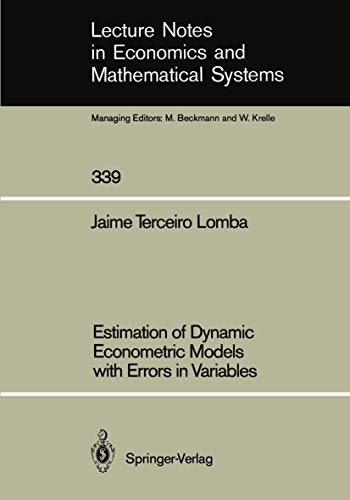Free Shipping in Australia
Proud to be B-Corp

# Estimation of Dynamic Econometric Models with Errors in Variables Jaime Terceiro Lomba\$181.59
Condition - New
Only 2 left

## Summary

A new procedure for the maximum-likelihood estimation of dynamic econometric models with errors in both endogenous and exogenous variables is presented in this monograph. A complete analytical development of the expressions used in problems of estimation and verification of models in state-space form is presented.

## Estimation of Dynamic Econometric Models with Errors in Variables Summary

### Estimation of Dynamic Econometric Models with Errors in Variables by Jaime Terceiro Lomba

A new procedure for the maximum-likelihood estimation of dynamic econometric models with errors in both endogenous and exogenous variables is presented in this monograph. A complete analytical development of the expressions used in problems of estimation and verification of models in state-space form is presented. The results are useful in relation not only to the problem of errors in variables but also to any other possible econometric application of state-space formulations.

1. Introduction.- 2. Formulation of Econometric Models in State-Space.- 2.1. Structural Form, Reduced Form and State-Space Form.- 2.2. Additional Remarks.- 3. Formulation of Econometric Models with Measurement Errors.- 3.1. Model of the Exogenous Variables.- 3.2. State-Space Formulation.- 4. Estimation of Econometric Models with Measurement Errors.- 4.1. Evaluation of the Likelihood Function.- 4.2. Maximization of the Likelihood Function.- 4.3. Initial Conditions.- 4.4. Gradient Methods and Identification.- 4.5. Asymptotic Properties.- 4.6. Numerical Considerations.- 4.7. Model Verification.- 5. Extensions of the Analysis.- 5.1. Missing Observations and Contemporaneous Aggregation.- 5.2. Temporal Aggregation.- 5.3. Correlated Measurement Errors.- 6. Numerical Results.- 7. Conclusions.- Appendices.- A. Kalman Filter and Chandrasekhar Equations.- A.1. Kalman Filter.- A.2. Chandrasekhar Equations.- B. Calculation of the Gradient.- C. Calculation of the Hessian.- D. Calculation of the Information Matrix.- E. Estimation of the Initial Conditions.- F. Solution of the Lyapunov and Riccati Equations.- F.1. Lyapunov Equation.- F.2. Riccati Equation.- G. Fixed-Interval Smoothing Algorithm.- References.- Author Index.

NLS9783540523581
9783540523581
3540523588
Estimation of Dynamic Econometric Models with Errors in Variables by Jaime Terceiro Lomba
New
Paperback
Springer-Verlag Berlin and Heidelberg GmbH & Co. KG
1990-04-04
121
N/A
Book picture is for illustrative purposes only, actual binding, cover or edition may vary.
This is a new book - be the first to read this copy. With untouched pages and a perfect binding, your brand new copy is ready to be opened for the first time